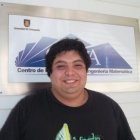﻿﻿ CI²MA - Publications | Undergraduate Thesis

# Undergraduate Thesis of Gonzalo RiveraCareerMathematical Civil Engineering, Universidad de Concepción
Enrollment Year2002
Senior Year2011
Thesis TitleNumerical Simulation of the Upper Respiratory Tract

#### Thesis Summary:

In the present work, a numerical model representing the snore emitted by a human being is described. In order to generate numerical simulations of how snoring occurs during respiration, it was necessary to generate a model that simulates the upper respiratory tract, along with the soft palate. For this purpose, a mechanical model analogous to that of  was considered, as shown in Figure 3, and the soft palate and upper respiratory tract were separately worked. Finally, the coupled problem was solved. Each of these stages is presented in 3 chapters. In the first chapter a numerical model was developed for the soft palate, for this it was supposed that it could be simulated by an elastic beam embedded at one end and free at the other. This was validated in . Since the elastic beam that was used for the model is of small length and thickness, it was determined that the theory that best fit this type of beams is that of Timoshenko. Following this, we proceeded to obtain the equations representing the vibration of the elastic beam for the steady and evolutionary case, we determined the variational formulation associated with these equations and we determined Analyzed this variational formulation. Finally, in this chapter some results are presented Numerals for the validation of the codes developed in Matlab. For this purpose, one-dimensional linear finite elements were used for the spatial part, with reduced integration for the cutter terms  and the Newmark method for the temporal part. In addition, it was observed that the evolutionary case of the elastic beam of Tymoshenko presents the same phenomenon as the stationary case, which is known as a numeric block of the method . The second chapter presents a numerical model to simulate the upper respiratory tract and the air circulating through it, for them the equations of an acoustic potential fluid were used, which are deduced from those of Navier- Stokes, then proceeded to determine its variational formulation and analyze it. Finally, a numerical example is presented for the validation of the codes developed in Matlab, which contains the implementation of the traditional finite element method (Galerkin) based on the [ 7] and Newmark's method for the temporal part. In the last chapter we proceeded to solve the problem coupled to the soft upper respiratory tract (acoustic fluid-elastic beam), for this was assumed the hypothesis of small fluctuations in speed and , Consequently, those of the pressure. A) Yes?? It can be assumed that the domain of the fluid does not change over time, however the variation of fluid pressures through the beam domain is that which produces the vibration of the latter, whereby the coupling between The models are given by the difference in pressure on both sides of the beam, which corresponds to the load acting on it.

Thesis Director(s) Rodolfo Rodríguez
Thesis Project Approval Date2011, April 12
Thesis Defense Date2011, December 12
Professional Monitoring
PDF TesisDownload Thesis PDF(No publications)

<< Back to list of Undergraduate Thesis.

 CI²MA, CENTER FOR RESEARCH IN MATHEMATICAL ENGINEERING, UNIVERSIDAD DE CONCEPCIÓN - MAILBOX 160-C, CONCEPCIÓN, CHILE, PHONE: +56-41-2661324